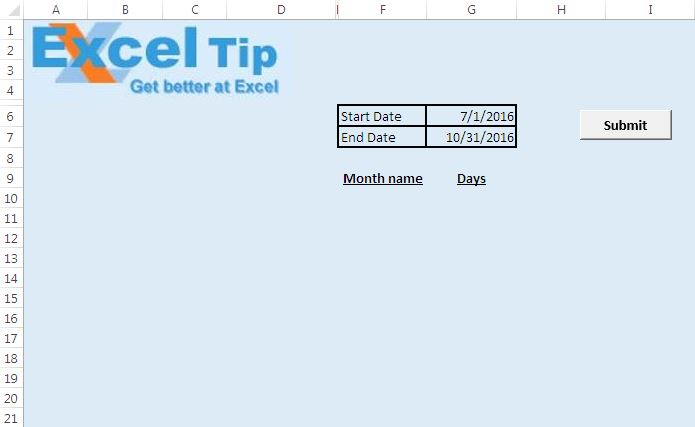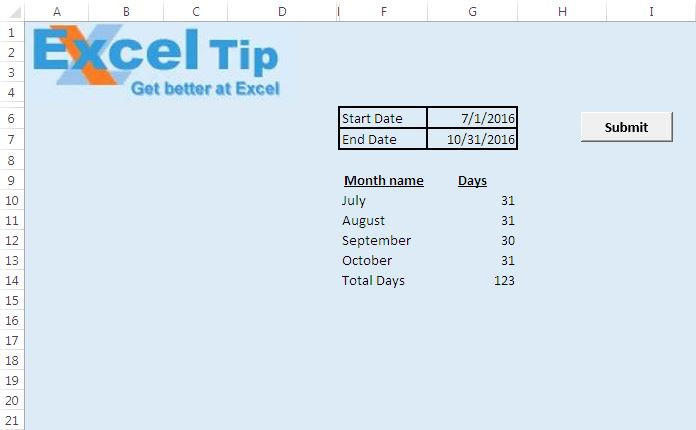# List and add days of months in a set period using VBA in Microsoft Excel

In this article, we will create a macro to list months between the defined period and also number of days in that particular month. It will also display the total number of days between the defined period.

We have created “DaysInPeriod” macro to list months and number of days in a month. Macro can be executed by clicking the “Submit” button.

Before running the macro, one has to provide input for start and end date. It takes value in cell “G6” as start date and value in cell “G7” as end date. Output will be displayed below in “F9” cell.On clicking the submit button, macro will display name of the month in column F and the number of days in that month in column G. The last row will show total number of days between the specified period.Logic explanation

In the macro, we start looping from starting date to the specified end date. While looping, we check for the last date of a month. If last date of a month is encountered, then the month name and number of days in that month is displayed in columns F and G. similarly, we also check for end date. When end date is encountered, entry for the last month and number of days in that month is displayed.

```Option Explicit

Sub DaysInPeriod()

Dim StartDate, EndDate As Date
Dim intRow As Integer, intDays As Integer

'Clearing previous content
Range("F10:G1048576").ClearContents

'Getting start and end date
StartDate = Range("G6")
EndDate = Range("G7")

'Initializing the variable to starting row number
intRow = 10

'Listing the months and number of days from starting date to end date
Do

intDays = intDays + 1

'Checking for last date of the month or when StartDate is equal to EndDate
If (Month(StartDate) <> Month(StartDate + 1)) Or StartDate = EndDate Then
'Inserting the month name
Cells(intRow, 6) = Format(StartDate, "mmmm")
'Inserting number of days in the month
Cells(intRow, 7) = intDays
'Moving to next row
intRow = intRow + 1
intDays = 0
End If

'Moving to next date
StartDate = StartDate + 1

Loop Until StartDate > EndDate

'Getting the sum in the last row
Cells(intRow, 6) = "Total Days"
Cells(intRow, 7) = Application.Sum(Range("G10:G" & intRow))

End Sub
```